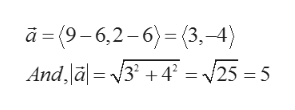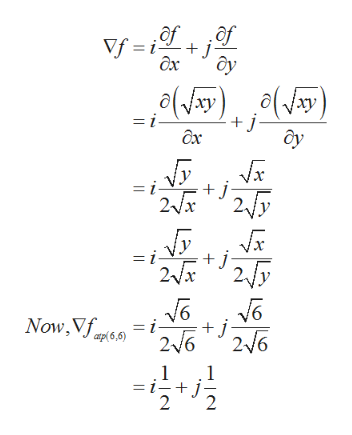Find the directional derivative of f(x, y) = (xy)^1/2 at P(6, 6) in the direction from P to Q(9, 2)

Question

Find the directional derivative of f(x, y) = (xy)^1/2

at P(6, 6) in the direction from P to Q(9, 2)

Step 1

The directional derivative (D.D) of function f at point P in the direction of vector a is given by,

Step 2

Here, the vector a is, vector in the direction from P(6,6) to Q(9,2).help_outlineImage Transcriptionclosea=(9-6,2-6)= (3.-4) And,a=3 +4 = 25 = 5 fullscreen
Step 3

Now, find the value of gradient of f at point P....help_outlineImage Transcriptioncloseof of Vf i j дх y o(y(ay) + j- = i- дх = i- j 2fry 2x = i- j Now,Vf = i + j 216 26 2 1 -+ j 2 1 fullscreen

Want to see the full answer?

See Solution

Want to see this answer and more?

Our solutions are written by experts, many with advanced degrees, and available 24/7

See Solution
Tagged in

Math